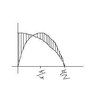# Area Under Curve: cos x = sin 2x, [0, pi/2]

• a.a

## Homework Statement

Find the area under bounded by y = cos x and y = sin 2x on the intervacl [0, pi/2]

## The Attempt at a Solution

cos x = sin 2x
2sin x cos x - cos x = 0

x = pi/6 and pi/2

shouldn't the intergral be: [ S(0,pi/6) cos x - sin 2x ] - [ S(pi/6,pi/2) sin 2x - cos x ]
I have [ S(0,pi/6) cos x - sin 2x ] - [ S(pi/6,pi/2) sin 2x + cos x ]
why is it sin 2x + cos x?

#### Attachments

•Untitled.jpg
4.7 KB · Views: 366
Since they ask for the area, and area is always positive, you don't need the negative multiple in front of your second integral (unless your text has explicitly defined area to mean signed area or the definite integral of a function over an interval).
I do not see why there is a sum instead of a difference in the second integral; it may be a typo.

so [ S(0,pi/6) cos x - sin 2x ] + [ S(pi/6,pi/2) sin 2x - cos x ] would be the area?

a.a said:
so [ S(0,pi/6) cos x - sin 2x ] + [ S(pi/6,pi/2) sin 2x - cos x ] would be the area?

Yep. :)